Examples

Chapter 8 Class 11 Sequences and Series
Serial order wise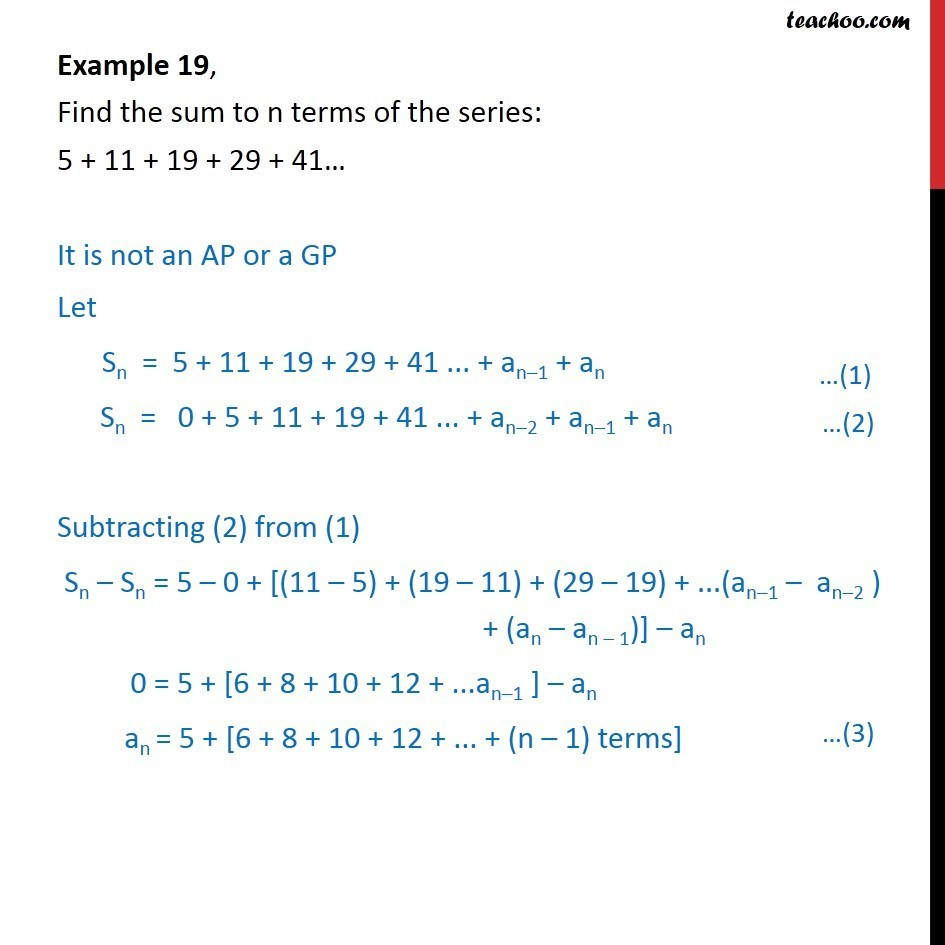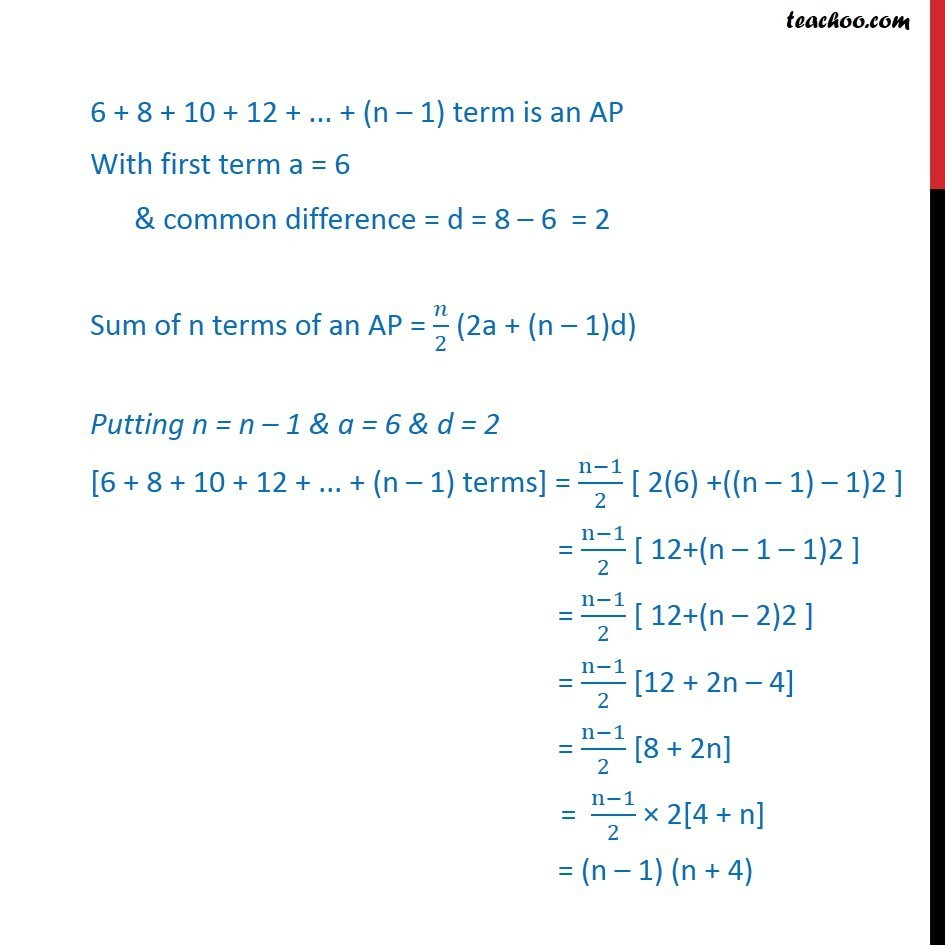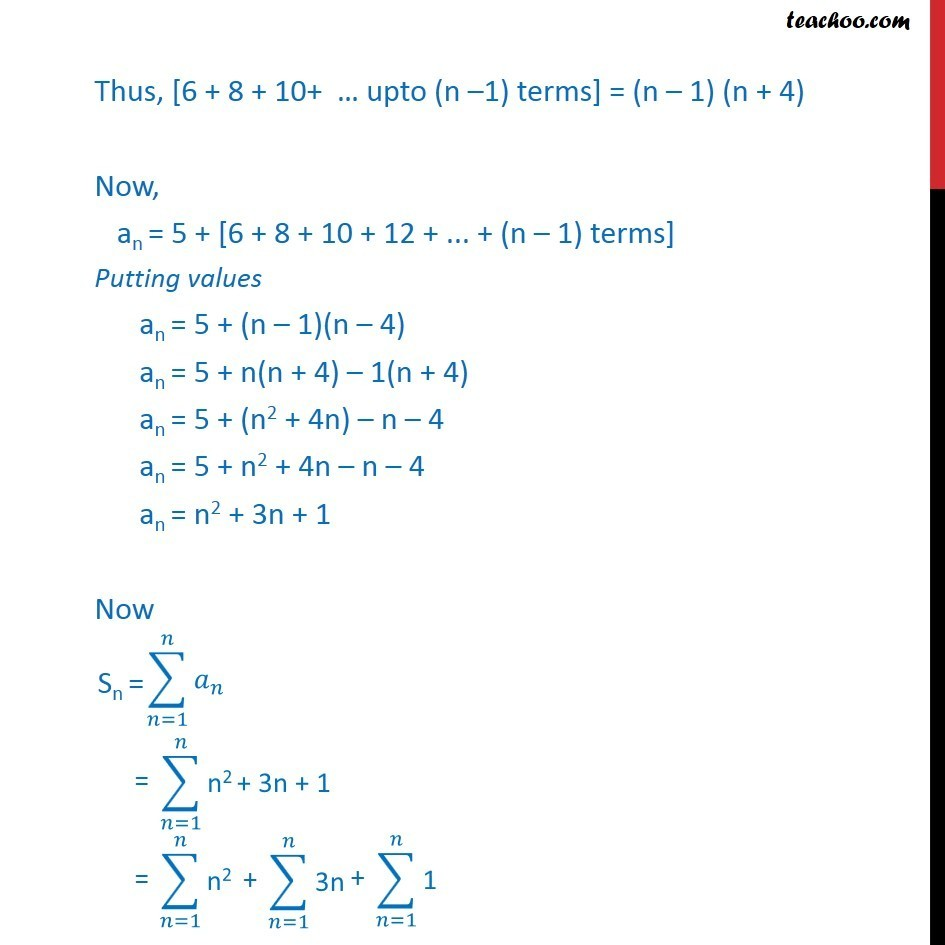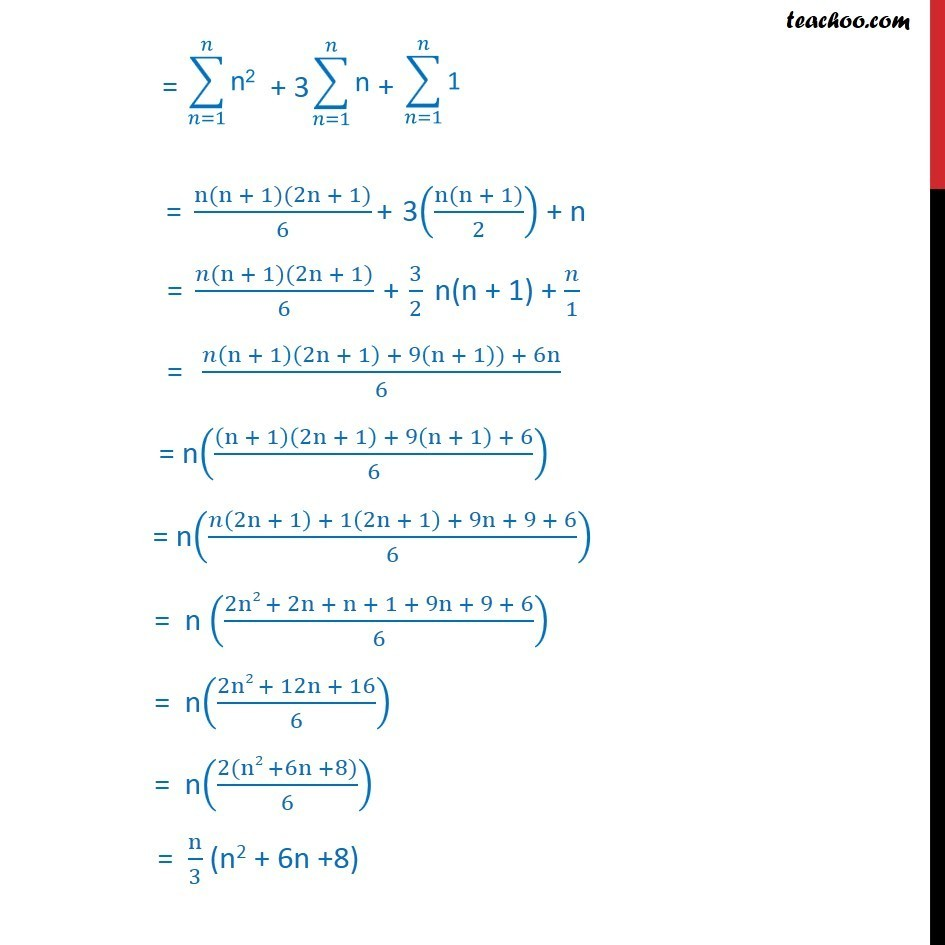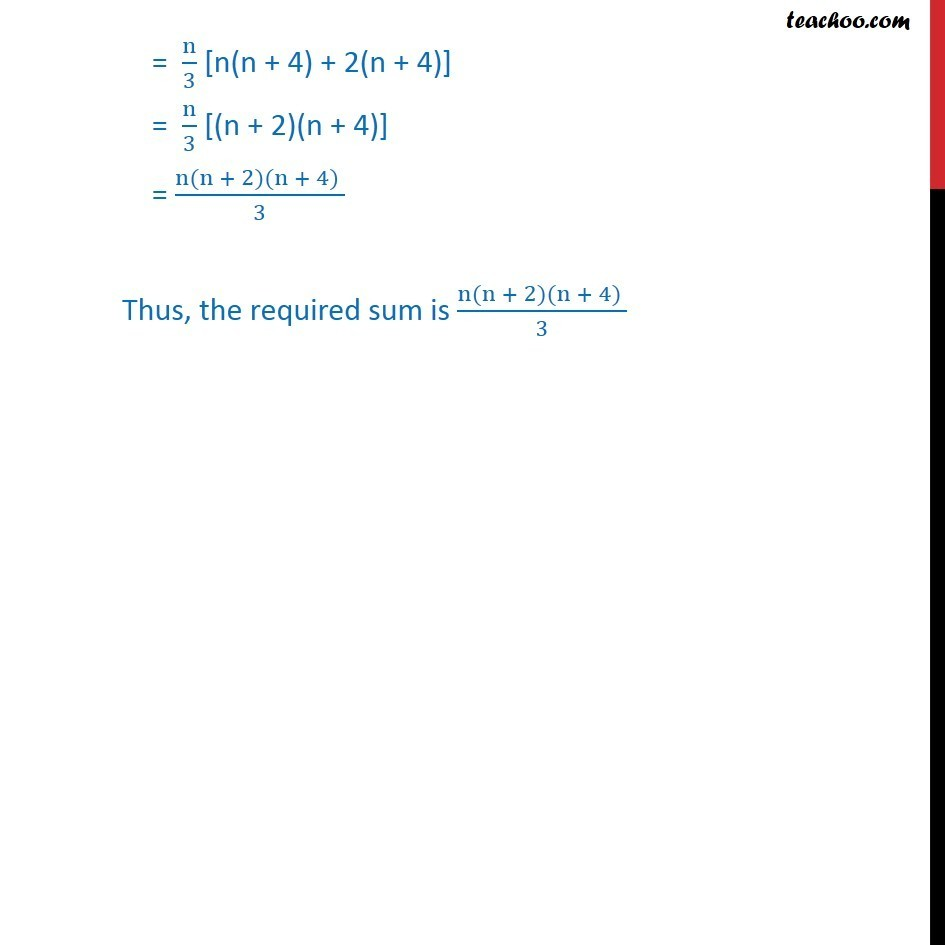Learn in your speed, with individual attention - Teachoo Maths 1-on-1 Class

### Transcript

Question 6, Find the sum to n terms of the series: 5 + 11 + 19 + 29 + 41… It is not an AP or a GP Let Sn = 5 + 11 + 19 + 29 + 41 ... + an–1 + an Sn = 0 + 5 + 11 + 19 + 41 ... + an–2 + an–1 + an Subtracting (2) from (1) Sn – Sn = 5 – 0 + [(11 – 5) + (19 – 11) + (29 – 19) + ...(an–1 – an–2 ) + (an – an – 1)] – an 0 = 5 + [6 + 8 + 10 + 12 + ...an–1 ] – an an = 5 + [6 + 8 + 10 + 12 + ... + (n – 1) terms] 6 + 8 + 10 + 12 + ... + (n – 1) term is an AP With first term a = 6 & common difference = d = 8 – 6 = 2 Sum of n terms of an AP = 𝑛/2 (2a + (n – 1)d) Putting n = n – 1 & a = 6 & d = 2 [6 + 8 + 10 + 12 + ... + (n – 1) terms] = (n−1)/2 [ 2(6) +((n – 1) – 1)2 ] = (n−1)/2 [ 12+(n – 1 – 1)2 ] = (n−1)/2 [ 12+(n – 2)2 ] = (n−1)/2 [12 + 2n – 4] = (n−1)/2 [8 + 2n] = (n−1)/2 × 2[4 + n] = (n – 1) (n + 4) Thus, [6 + 8 + 10+ … upto (n –1) terms] = (n – 1) (n + 4) Now, an = 5 + [6 + 8 + 10 + 12 + ... + (n – 1) terms] Putting values an = 5 + (n – 1)(n – 4) an = 5 + n(n + 4) – 1(n + 4) an = 5 + (n2 + 4n) – n – 4 an = 5 + n2 + 4n – n – 4 an = n2 + 3n + 1 Now = (n(n + 1)(2n + 1))/6 + 3((n(n + 1))/2) + n = (𝑛(n + 1)(2n + 1))/6 + 3/2 n(n + 1) + 𝑛/1 = (𝑛(n + 1)(2n + 1) + 9(n + 1)) + 6n)/6 = n(((n + 1)(2n + 1) + 9(n + 1) + 6)/6) = n((𝑛(2n + 1) + 1(2n + 1) + 9n + 9 + 6)/6) = n ((2n2 + 2n + n + 1 + 9n + 9 + 6)/6) = n((2n2 + 12n + 16)/6) = n((2(n2 +6n +8))/6) = n/3 (n2 + 6n +8) = n/3 [n(n + 4) + 2(n + 4)] = n/3 [(n + 2)(n + 4)] = (n(n + 2)(n + 4) )/3 Thus, the required sum is (n(n + 2)(n + 4) )/3